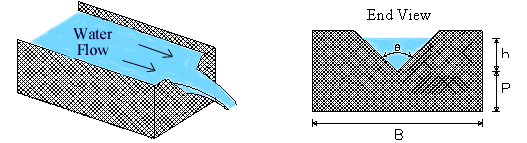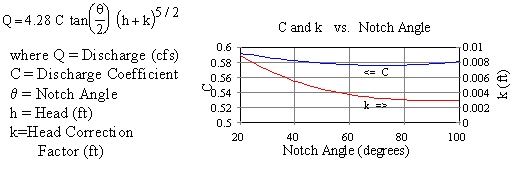V-Notch (Triangular) Weir Calculator

Discharge and Head Calculations, Equations, and Guidelines for water flow measurement in streams and channels.

 Solve for: Discharge Head Discharge, Q: gal/min (gpm) gal/day (gpd) Million gal/day (MGD) ft3/s (cfs) m3/s m3/day Head, h: inch ft cm m Notch Angle, θ: degrees radians Discharge Coefficient, C: Head Correction Factor, k: ft © 2014 LMNO Engineering, Research, and Software, Ltd. http://www.LMNOeng.comUnits in V-notch weir calculation: ft3=cubic foot, gal=US gallons, m3=cubic meter, mm=millimeter, s=second

Topics for V-notch weir: Introduction    Equations     Installation Guidelines and Equation Applicability    Error Messages    References

Introduction
Weirs are typically installed in open channels such as streams to determine discharge (flowrate). The basic principle is that discharge is directly related to the water depth above the crotch (bottom) of the V; this distance is called head (h). The V-notch design causes small changes in discharge to have a large change in depth allowing more accurate head measurement than with a rectangular weir.

Triangular Weir (V-Notch Weir) Equations
V-notch weir equations have become somewhat standardized. ISO (1980), ASTM (1993), and USBR (1997) all suggest using the Kindsvater-Shen equation, which is presented below from USBR (1997) for Q in cfs and heights in ft units. All of the references show similar curves for C and k vs. angle, but none of them provide equations for the curves.   To produce automated calculations, LMNO Engineering used a curve fitting program to obtain the equations which best fit the C and k curves. Our equations are shown below.  The graph shown is from our fits. If you compare it to the graphs shown in the references, it looks nearly identical which implies that our fits are very good.C = 0.607165052 - 0.000874466963 θ  +  6.10393334x10-6 θ2
k (ft.) = 0.0144902648 - 0.00033955535 θ  + 3.29819003x10-6 θ2  - 1.06215442x10-8 θ3
where θ is the notch angle in degrees

Installation Guidelines and Equation Applicability                     Top of Page
USBR (1997) suggests using the V-notch weir equations for the following conditions:

Head (h) should be measured at a distance of at least 4h upstream of the weir.

It doesn't matter how thick the weir is except where water flows over the weir through the "V." The weir should be between 0.03 and 0.08 inches (0.8 to 2 mm) thick in the V. If the bulk of the weir is thicker than 0.08 inch, the downstream edge of the V can be chamfered at an angle greater than 45o (60o is recommended) to achieve the desired thickness of the edges. You want to avoid having water cling to the downstream face of the weir.

Water surface downstream of the weir should be at least 0.2 ft. (6 cm) below the bottom of the V to allow a free flowing waterfall.

Measured head (h) should be greater than 0.2 ft. (6 cm) due to potential measurement error at such small heads and the fact that the nappe (waterfall) may cling to the weir.

The equations have been developed for h<1.25 ft. (38 cm) and h/P<2.4.

The equations have been developed for fully contracted V-notch weirs which means h/B should be ≤ 0.2.

The average width of the approach channel (B) should be > 3 ft. (91 cm).

The bottom of the "V" should be at least 1.5 ft. (45 cm) above the bottom of the upstream channel.

If your weir does not achieve some of the above criteria, you may have a "partially contracted V-notch weir" where h/B needs only to be ≤ 0.4, the bottom of the "V" only needs to be 4 inch (10 cm) above the bottom of the upstream channel, the approach channel only needs to be 2 ft. (61 cm) wide, and h can be up to 2 ft. (61 cm) instead of 1.25 ft. (38 cm). Partially contracted weirs use a different graph for C which is a function of h/P and P/B and is only valid for a notch angle of 90o. In the graph (not shown - see USBR, 1997), C varies from 0.576 to 0.6; whereas, for a fully contracted 90o notch, C is 0.578 from our graph shown above. Our calculation does not account for partially contracted weirs, but for most practical purposes the difference in C is inconsequential.

Error Messages given by V-notch weir calculation                                Top of Page
"All inputs must be positive". This is an initial check of user input.
"Angle out of range". The notch angle must be between 20o and 100o (0.35 and 1.75 radians) for the equations to be valid.
"Infeasible input". Input results in a negative head due to the compiler's machine precision. Occurs if head is being computed and a very low Q is entered (e.g. 1.0e-20).

References
ASTM. (1993). American Society for Testing and Materials. ASTM D5242. Standard method for open-channel flow measurement of water with thin-plate weirs. 1993. Available from Global Engineering Documents at http://global.ihs.com

ISO. (1980). International Organization of Standards. ISO 1438/1-1980(E). Water flow measurement in open channels using weirs and venturi flumes - Part 1: Thin plate weirs. 1980. Available from Global Engineering Documents at http://global.ihs.com

USBR. (1997). U.S. Department of the Interior, Bureau of Reclamation. Water Measurement Manual. 3ed. Available from http://www.usbr.gov/tsc/techreferences/mands/wmm/index.htm .

LMNO Engineering, Research, and Software, Ltd.
7860 Angel Ridge Rd.   Athens, Ohio  45701  USA   Phone: (740) 707-2614
LMNO@LMNOeng.com    http://www.LMNOeng.com

To:

Related calculations:

Weirs:
Rectangular
Cipoletti

End Depth Method: Circular
Rectangular
Triangular

Unit Conversions

Register